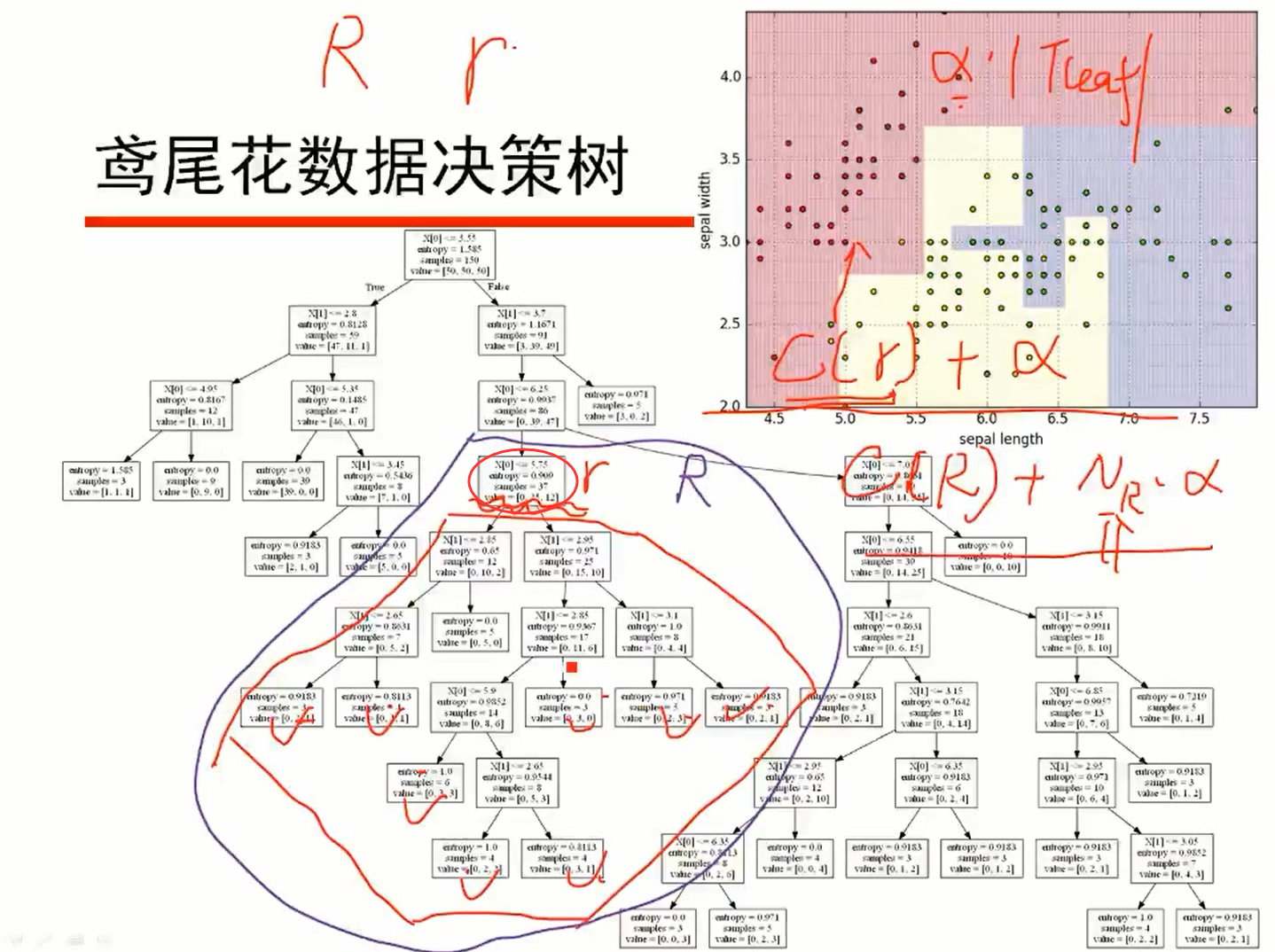# 决策树剪枝算法原理

## 算法目的：

• 同样层数的决策树，叶结点的个数越多就越复杂；同样的叶结点个数的决策树，层数越多越复杂。
• 剪枝前相比于剪枝后，叶结点个数和层数只能更多或者其中一特征一样多，剪枝前必然更复杂。
• 层数越多，叶结点越多，分的越细致，对训练数据分的也越深，越容易过拟合，导致对测试数据预测时反而效果差。

## 模型损失函数

1. 变量预定义：$|T_{leaf}|$表示树T的叶结点个数，t表示树T的叶结点，同时，$N_t$表示该叶结点含有的样本点个数，其中属于k类的样本点有$N_{tk}$个，K表示类别的个数，$H_t(T)$为叶结点t上的经验熵，$\alpha≥0$为参数。
显然有$N_t=\displaystyle \sum^{K}_{k=1} {N_{tk}}$
2. 经验熵：$H_t(T) = -\displaystyle \sum_k^K \frac {N_{tk}} {N_t} \log \frac {N_{tk}}{N_t}$
3. 评价函数即损失函数：$C(T)=\displaystyle \sum^{|T_{leaf}|}_{t=1} {N_tH_t(T)}$
4. 避免过拟合修正后的评价函数：$C_a(T)=\displaystyle \sum^{|T_{leaf}|}_{t=1} {N_tH_t(T)+\alpha|T_{leaf}|}$
损失函数简化形式：$C_a(T)=C(T)+\alpha|T_{leaf}|$
• 经验熵反映了一个叶结点中的分类结果的混乱程度。经验熵越大，说明该叶结点所对应的分类结果越混乱，也就是说分类结果中包含了较多的类别，表明该分支的分类效果较差。
• 评价函数其实是求叶结点的经验熵期望。用$N_t$给经验熵加权的依据是叶子节点含有的样本个数越多，其分类效果或混乱程度越占主导，相当于求了期望，可以更好的描述分支前后的关系。例如设一个结点r有n个样本，其组成是第i类有$n_i$个样本，在分了几个孩子结点后各个叶结点的成分仍保持原比例，记新的子树为R，可以计算得出评价函数$C(r)=C(R)$，即在随机分组后不会有任何分类效果的改进。
• 评价函数越小越好。熵的期望和熵一样，越小越好。所以，评价函数越大，说明模型的分类效果越差，因而又称损失函数。
• 修正项$\alpha|T_{leaf}|$是基于复制度的考虑。如上面提到的情况，r与R其实是一样的，没有进行任何分类的处理，但是我们仍然觉得r更好，原因在于R的复杂度更高。加了此修正项后具有现实的意义：如果$\alpha=0$表示未剪枝的完全树损失更小（熵更小的占主导地位），如果$\alpha->∞$表示剪枝到只剩根结点更好（叶结点个数占主导地位）。
修正项$\alpha|T_{leaf}|$可以避免过拟合。修正项$\alpha|T_{leaf}|$考虑到了复杂度，$\alpha$值设置得好可以避免出现完全树和根结点这类的极端情况，因此可以避免过拟合。
纯结点的经验熵$H_p=0$最小，即设$N_{tj}=N_t$则有$H_p=-(1\log 1+\displaystyle \sum_{k=1...K且k≠j} {0})=0$
均结点的经验熵$H_u=\log K$最大，即$H_p=-\displaystyle \sum^{K}_{k=1} {\frac{1}{K}\log\frac{1}{K}}=\log K$

## 剪枝类型：

• 预剪枝是在决策树生成过程中，对树进行剪枝，提前结束树的分支生长。
• 后剪枝是在决策树生长完成之后，对树进行剪枝，得到简化版的决策树。

• 作为叶结点或作为根结点需要含的最少样本个数
• 决策树的层数
• 结点的经验熵小于某个阈值才停止

1. 如何由$T_i$剪枝得到$T_{i-1}$
如图• $C_\alpha(r)=C(r)+\alpha$
• $C_\alpha(R)=C(R)+N_R*\alpha$，其中$N_R$为子树R的叶子结点数
• 一般地有$C(r)>=C(R)$， 我们可以令$C_\alpha(r)=C_\alpha(R)$求出$\alpha=\frac{C(r)-C(R)}{N_R-1}$
• 当我们把所有的非叶结点的$\alpha$求出来后比较它们的$\alpha$值，在最小的$\alpha$所在结点处剪枝。
为什么要在最小的$\alpha$所在结点处剪枝：设给定一$\alpha$来求两个结点$r_1$$r_2$处的损失函数$C_\alpha(r_1)$$C_\alpha(r_2)$以判定是否适合继续分支，且使得给定的$\alpha$介于它们自己的阈值参数$\alpha_1$$\alpha_2$之间，假设$\alpha_1$<$\alpha$<$\alpha_2$。则
对于$r_1$来说，$\alpha_1$<$\alpha$$\alpha$给大了，参数占主导，剪枝能使得叶结点数（即参数的系数）变小，剪枝能使得$C_\alpha(r_1)$较小，剪枝更好；
对于$r_2$来说，$\alpha$<$\alpha_2$$\alpha$给小了，$C(r_2)-C(R_2)$的值占主导，即
$C_\alpha(r)- C_\alpha(R)$
$=C(r)+\alpha-C(R)-N_R*\alpha$
$= [C(r_2)-C(R_2)]+[1-N_R]*\alpha$
不剪枝能使得叶结点数（即参数的系数）更大，$C_\alpha(r)- C_\alpha(R)$更接近0，剪枝更好。
我们看出参数阈值较小的$r_1$处剪枝更好，因此要在最小的$\alpha$所在结点处剪枝。
1. $T_i$剪枝得到$T_{i-1}$后，可以得出$T_0->T_1->T_2->...->T_n$，但不一定每次剪枝都能使得损失函数降低，可以对每一个$T_i$算出其损失函数进行比较，取损失函数值最小的那棵决策树。